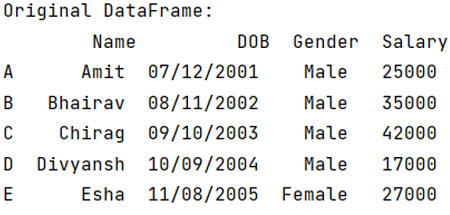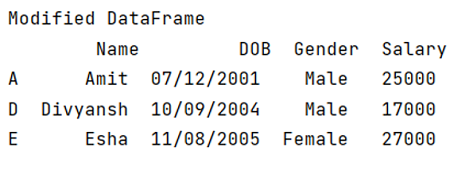# How to drop a list of rows from Pandas DataFrame?

Given a DataFrame, we have to drop a list of rows from it. By Pranit Sharma Last updated : September 19, 2023

Rows in pandas are the different cell (column) values which are aligned horizontally and also provides uniformity. Each row can have same or different value. Rows are generally marked with the index number but in pandas we can also assign index name according to the needs. In pandas, we can create, read, update and delete a column or row value.

## Problem statement

Given a DataFrame, we have to drop a list of rows from it.

## Dropping a list of rows from Pandas DataFrame

For this purpose, we will use pd.DataFrame.drop() method. This method is used to remove a specified row or column from the pandas DataFrame. Since rows and columns are based on index and axis values respectively, by passing index or axis value inside DataFrame.drop() method we can delete that particular row or column. Below is the syntax:

### Syntax

```DataFrame.drop(
labels=None,
axis=0,
index=None,
columns=None,
level=None,
inplace=False,
errors='raise'
)

# or
df.drop(axis=None)  # deletes a specified column.
df.drop(index=None) # deletes a specified row.
```
Note

To work with pandas, we need to import pandas package first, below is the syntax:

```import pandas as pd
```

Let us understand with the help of an example:

## Python program to create and print pandas dataFrame

```# Importing pandas package
import pandas as pd

# Creating a Dictionary
dict = {
'Name':['Amit','Bhairav','Chirag','Divyansh','Esha'],
'DOB':['07/12/2001','08/11/2002','09/10/2003','10/09/2004','11/08/2005'],
'Gender':['Male','Male','Male','Male','Female'],
'Salary':[25000,35000,42000,17000,27000]
}

# Creating a DataFrame, since we need to drop a list of row
# and we know that rows have particular indexes
# so we will assign some names to each row for
# better understanding
df = pd.DataFrame(dict, index=['A','B','C','D','E'])

# Display original DataFrame
print("Original DataFrame:\n",df,"\n")
```

### OutputNow, we will drop a list of rows where index = 'B' and 'C'.

## Python program to drop a list of rows from Pandas DataFrame

```# Dropping rows with index B and C
result = df.drop(['B','C'])

# Display Result
print("Modified DataFrame\n",result)
```

### Output